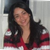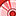# QlikView App Dev

Discussion Board for collaboration related to QlikView App Development.

Announcements
Customer & Partners, DEC. 9, 11 AM ET: Qlik Product & Strategy Roadmap Session: Data Analytics REGISTER NOW
cancel
Showing results for
Did you mean:Contributor II

## Count Distinct IF (Conditions based on variables)

I know you've already posted similar cases and gotten answers. Based on them I have been able to solve some issues that I had but still present one.

I have to count some codes of the current year and in the corresponding month when the difference between two dates is 0.

This is the code that has issues:

COUNT(DISTINCT(IF(\$(DIFF_YEAR) = 0 AND [YEAR] = {'CYTD'} AND MONTH = \$(=MONTH_NO), CODE)))

When I use the following instruction it works:
COUNT(DISTINCT(IF(\$(DIFF_YEAR) = 0, CODE)))

But when I add AND [YEAR] = {'CYTD'}  and MONTH = \$(=MONTH_NO). Does not show results.

Labels (1)
• ### Count Distinct if

1 Solution

Accepted SolutionsContributor II
Author

Hi guys,

Thank you for your help. I'd like to share with you that I got the info that I needed so far.

I used this sentence and it's working:

COUNT( DISTINCT( IF( \$(DIFF_YEAR) = 0 AND YEAR = 'CYTD' AND MTH_NO = \$(=MONTH_NO), INFORCE_POL_NO)))

I was checking the month values when you (Kush) asked me and I found that I was taking a bad field MONTH and I have to take  MTH_NO.

10 RepliesSpecialist II

If CYTD and MONTH_NO are variables,

COUNT(DISTINCT(IF(\$(DIFF_YEAR) = 0 and [YEAR] = \$(CYTD) and MONTH=\$(MONTH_NO), CODE)))

Also, if you put single quotes around \$(CYTD) in the IF statement, you would also put single quotes around [YEAR].  I test variables by displaying them in a text box to ensure I know what I'm comparing.Contributor II
Author

Hi,

I'm working in this issue, and the sentence is working but, only with two conditions:

COUNT(DISTINCT(IF(\$(DIFF_YEAR) = 0 AND YEAR = 'CYTD', INFORCE_POL_NO))))

When I added this condition: AND MONTH = \$(=MONTH_NO). It didn't work.MVP

try below

COUNT(DISTINCT(IF(\$(DIFF_YEAR) = 0 AND [YEAR] = 'CYTD' AND MONTH = \$(MONTH_NO), CODE)))Contributor II
Author

Hi,

I tried that but, when I used MONTH = \$(MONTH_NO) the sentence does not recognize it. When I use MONTH = \$(=MONTH_NO) the sentence recognize the month.MVP

MONTH_NO is field? What values MONTH & MONTH_NO have?Contributor II
Author

Hi Kush,

MONTH is a field and MONTH_NO is a variable containing the last month of a report I have uploaded. e.g It is currently 6 because I was working with the June data.MVP

I can see that variable is having blank value. Can you check the variable value in KPI object? What is expression used in that variable?Contributor II
Author

Hi Kush,

When I used MONTH = \$(MONTH_NO) I didn't get the variable value, the value is in blank; however, when I use MONTH = \$(=MONTH) I get the value. In the following example you can check itNow, my issue is because when I use two conditions (DIFF_YEAR & YEAR) I have results but, when I use MONTH as well I did't get it.MVP

Check if your month field has a number value? try  with quotes '\$(=MONTH_NO) ' or '\$(MONTH_NO) 'Community Browser